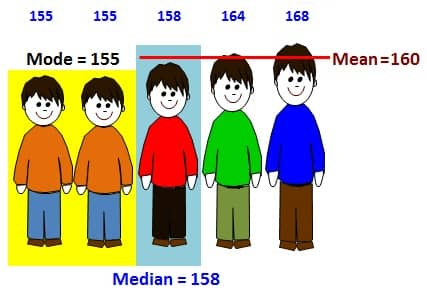The K5 Learning Blog urges parents to be pro-active in helping their children reach their full academic potential.

K5 Learning
provides an online reading and math program for kindergarten to grade 5 students.What’s the Difference between Mean, Median and Mode?Mean, median and mode are three kinds of ‘averages’.

The Mean

The "mean" is the "average" you're used to, where you add up all the numbers and then divide by the number of numbers.

For example - find the mean of the following list of numbers:

13, 18, 13, 14, 13, 16, 14, 21, 13

The mean is the usual average:

(13 + 18 + 13 + 14 + 13 + 16 + 14 + 21 + 13) ÷ 9 = 15

The Median

The “median” is the middle item after the data is organized from the least to the greatest. Exactly half of the data is before the median, and the other half is after.

For example – find the median of the following list of numbers:

8, 9, 10, 10, 10, 11, 11, 11, 12, 13

You'll need to average the fifth and sixth numbers to find the median:

(10 + 11) ÷ 2 = 21 ÷ 2 = 10.5

The Mode

The “mode” is the most commonly occurring data item within the set of numbers.

If no item occurs more often than others, there is no mode.

For example, the set of numbers -  1, 3, 5 8, 9, 10 has no mode.

If two (or three, four, etc.) items occur equally often, there are that many modes.

For example, the set of numbers - 3, 3, 6, 6, 7, 8, 8, 10 has three modes: 3, 6, and 8.

Sometimes, there is just one mode.

For example, in the set of numbers - 8, 9, 10, 10, 11, 11, 11, 12, 13. The mode is 11.

The only hard part of finding the mean, median, and mode is keeping straight which "average" is which.

Just remember the following:
mean: regular meaning of "average"
median: middle value
mode: most often

For practice, we have some statistics and data & graphs workbooks that include ‘mean, median and mode’ in our bookstore.